Download the eSaral app and start learning from Kota's top IITians and doctors.

# Semiconductor Class 12 Important Questions-Fully Solved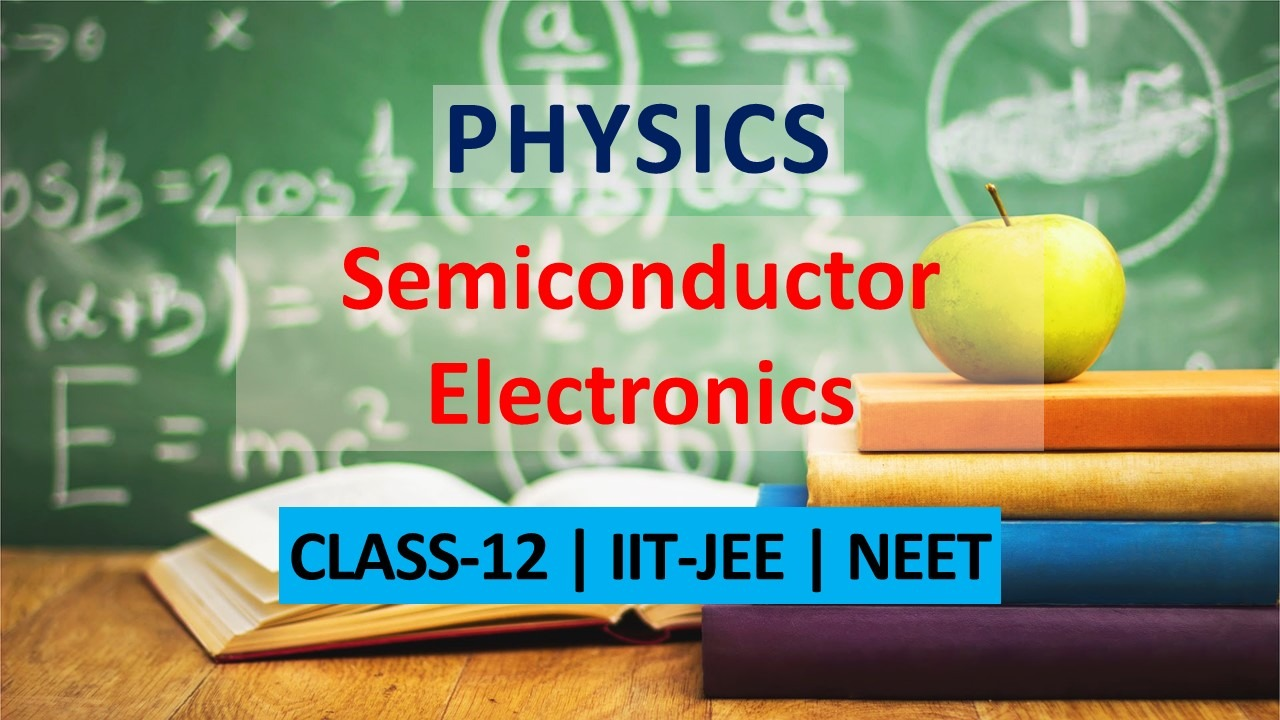Get important questions of Semiconductor Class 12 for Boards exams. View the Physics question bank for Class 11 & 12. These important questions will play significant role in clearing concepts of Semiconductor chapter. This question bank is designed keeping NCERT in mind and the questions are updated with respect to upcoming Board exams. You will get here all the important questions for class 12 Physics chapters. Click Here for Detailed Chapter-wise Notes of PHYSICS for Class 12th, JEE & NEET.
Q. Draw the voltage current characteristic of a zener diode
Ans.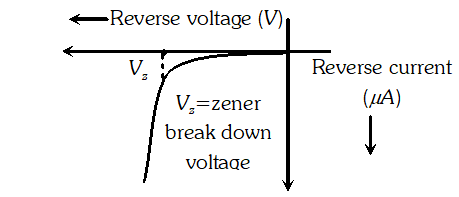Q. In the following circuits which one of the two diodes is forward biased and which is reverse biased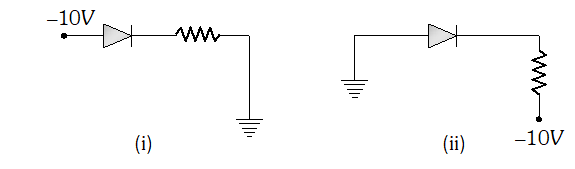Ans. (i) Reverse biased (ii) Forward biased.
Q. In half wave rectifier, what is the output frequency if the input frequency is 50 Hz. What is the output frequency of a full wave rectifier for the same input frequency. [NCERT]
Ans. The output ripple frequency is 50 Hz for half wave rectifier and 100 Hz for full wave rectifier
Q. Which one of the transistors p-n-p and n-p-n is more useful and why? [NCERT]
Ans. n-p-n is more useful because in it the current carriers are mainly electrons which are more speedy carriers than holes (the current carriers in p-n-p)
Q. Distinguish between N-type and P-type semiconductors on the basis of energy band diagrams
Ans. N-type semiconductor : Electrons are majority charge carriers in conduction band and holes are minority charge carriers in valance band. Donor energy level lies just below the conduction band. P-type semiconductor : Electrons are minority charge carriers in conduction band and holes are majority charge carriers in valence band. Impurity (acceptor) energy level lies just above the valence band.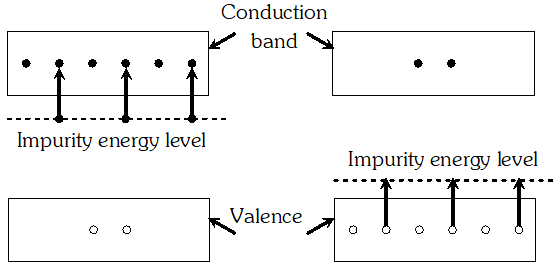Q. Draw a circuit diagram to show how a photo diode is biased. Draw it’s characteristic curves for two different illumination intensities
Ans.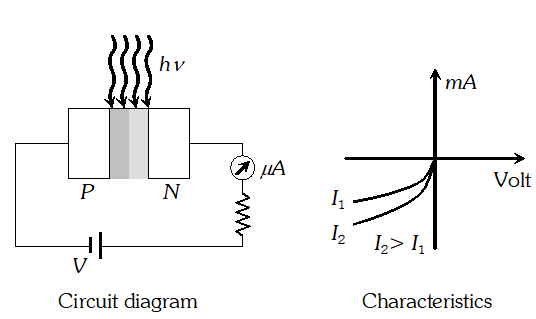Q. What do the acronyms ‘LASER’ and ‘LED’ stand for? Name the factor which determines (i) Frequency, and (ii) Intensity of light emitted by LED.
Ans.  LASER stands for light amplification by stimulated emission of radiation. LED stands for light emitting diode (i) The frequency of light emitted by an LED depends on the band gap of the semiconductor used in LED. (ii) The intensity of light depends on the doping level of the semiconductor used.
Q. Explain how the width of depletion layer in a p-n junction diode changes when the junction is (i) forward biased (ii) reverse biased.
Ans. When a p-n junction is forward biased, the majority charge carries flow towards the junction, the width of the depletion layer decreases, as shown on next page. (iii) Semiconductors : In semiconsuctors, a finite but small band gap $\left(E_{g}<3 e V\right)$ exists between valence band and conduction band. Because of the small band gap, at room temperature some electrons from valence band can acquire enough energy to cross the energy gap and enter the conduction band. For Ge band gap is 0.7 eV and for silicon it is about 1.1 eV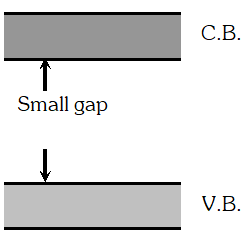Q. Explain how an intrinsic semiconductor can be converted into (i) N-type and (ii) P-type semiconductor. Give one example of each and their energy band diagrams.
Ans. (i) N-Type Semiconductor : Suppose a pure semiconductor say silicon in which each atom has four valence electrons is doped with pentavalent impurity say Arsenic (As). The four of the five valence electrons of impurity atom will form covalent bonds by sharing the electrons with the adjoining four atoms of silicon, while the fifth electron is very loosely bound with the parent impurity atom and is comparatively free to move. Thus each impurity atom donate one free electron to the crystal, that is why impurity is called donor impurity. On giving one electron, the donor atom becomes positively charged. However, the crystal remains electrically neutral as a whole. The semiconductor so formed is called N-type semi conductor. In N-type semiconductors, electrons are majority charge carriers and holes are minority charge carriers.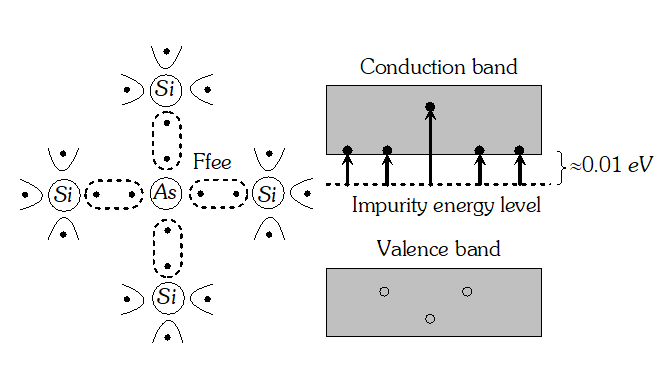(ii) P-type semiconductor : When a pure semiconductor say silicon (having four valence electron) doped with a trivalent impurity say Aluminium (having three valence electron) the impurity atom will replace the Si atom as shown in figure. The three valence electrons of impurity atom will form covalent bonds by sharing the electrons of the adjoining atoms of Si while there will be one incomplete covalent bond with a neighbouring Si atom due to the deficiency of an electron. This deficiency is completed by taking an electron from one of the Si-Si bonds. This makes Al ionised (negatively charged) and creates hole. This type of semi conductor is called P-type semiconductor. In P-type semiconductor, impurity is called acceptor impurity and the net charge of the crystal as a whole is zero. Holes are majority charge carriers and electrons are minority charge carriers in such type of semiconductors.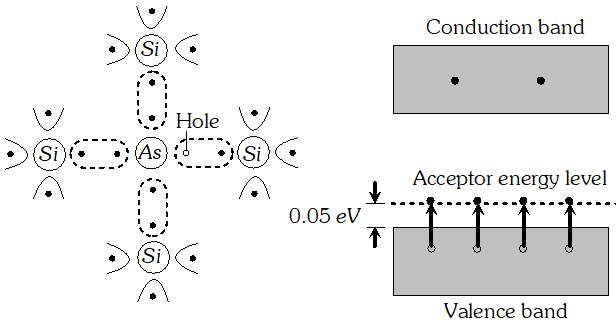Q.  What is zener diode? how is it symbolically represented? With the help of a circuit diagram, explain the use of zener diode as a voltage stabilizer
Ans.  Zener diode : The specially designed junction diodes, which can operate in the reverse breakdown voltage region continuously without getting damaged, are called zener diodes.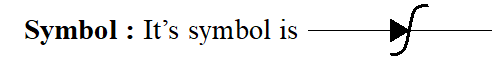As a voltage stabilizer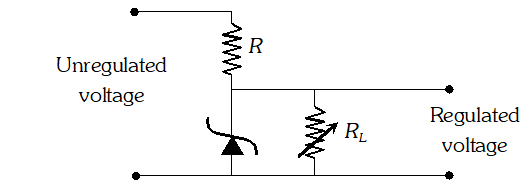The unregulated dc voltage (filtered output of a rectifier) is connected to the zener diode through a series resistance R such that the zener diode is reverse biased. If the input voltage increases, the current through R and zener diode also increases. This increases the voltage drop across R without any change in the voltage across the zener diode. This is because in the breakdown region, zener voltage remains constant even through the current through the zener diode changes. Thus any increase or decrease in input voltage results in increase or decrease of the voltage drop across R without any change in voltage across the zener diode. Thus the zener diode acts as a voltage regulator.
Q. Explain briefly, with the help of circuit diagram, how V-I characteristics of a p-n junction diode are obtained in (i) Forward bias, and (ii) Reverse bias. Draw the shape of the curves obtained
Ans.  Figures below shows a forward biased p-n junction and its voltage-current graph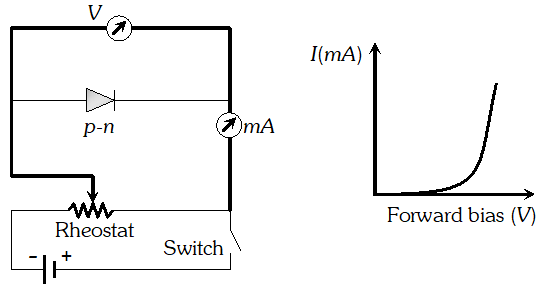Figure below show a reverse biased p-n junction and its voltage current graph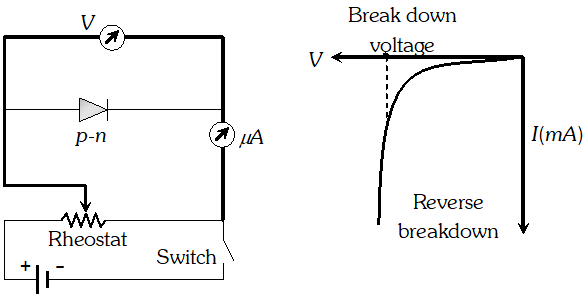In both cases, the battery is connected to the p-n junction through a rheostat so that the applied voltage can be changed. For different values of voltages, the value of current in noted. A graph is plotted between V and I.
Q. Explain (i) Forward biasing (ii) Reverse biasing of a P-N junction diode. With the help of a circuit diagram, explain the use of this device as a half-wave rectifier
Ans.  Forward biasing : A P-N junction is said to be forward biased if the positive terminal of the external battery is connected to the P-side and the negative terminal to the n-side of P-N junction. In forward biasing, potential barrier height is reduced and width of depletion layer decreases. In forward biasing the resistance of P-N junction is low to the flow of current.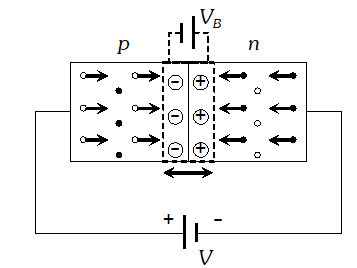Reverse Biasing : A P-N junction is said to be reverse biased if the positive terminal of the external battery is connected to n-side and the negative terminal to p-side. In reverse biased the height of barrier potential and width of depletion region increases. The resistance of p-n junction is high to the flow of current when reverse biased.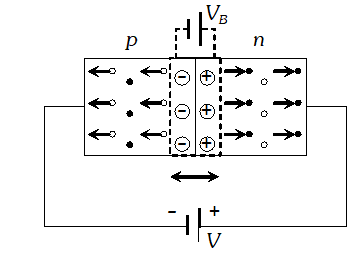A half-wave rectifier consists of a single diode, as shown in the circuit diagram. The secondary of the transformer gives the desired ac voltage across A and B. When the voltage at A is positive, the diode is forward biased and it conducts current. When A is negative, the diode is reverse biased and it does into conduct current. Thus we get output across RL during positive half-cycles only. The output is unidirectional but varying.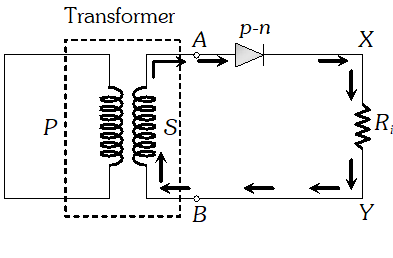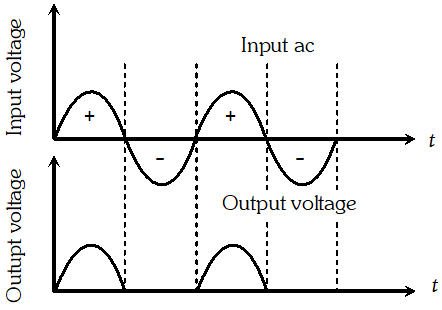Q. Can we take one slab of p-type semiconductor and physically join it to another n-type semiconductor to get p-n junction [NCERT]
Ans. No! Any slab, howsoever flat, will have roughness much larger than the inter atomic crystal spacing (–2 to 3Å) and hence continuos contact at the atomic level will not be possible. The junction will behave as a discontinuity for the flowing charge carriers.
Q. A transistor is a temperature sensitive device. Explain [NCERT]
Ans. In a transistor, free electrons and holes are the charge carriers and are responsible for the current through the transistor as well as in the external circuit. If temperature rises, more covalent bonds are broken in the semi-conducting material of the transistor giving rise to additional free electrons and holes. The results in larger current in the transistor and in the external circuit. The effect may be cumulative resulting in excessive heat and ultimately in permanent damage of transistor structure.
Q. Explain with the help of a labelled circuit diagram the use of a transistor as an oscillator
Ans. Circuit diagram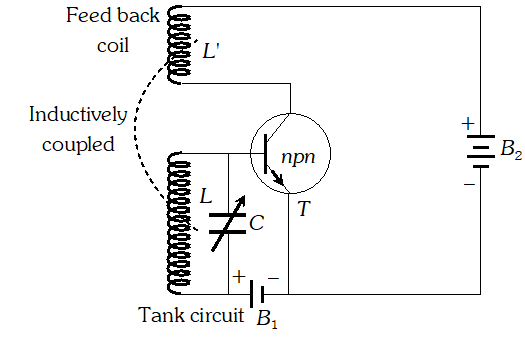$L, L^{\prime} \rightarrow$ Inductive coils, $C \rightarrow$Variable capacitor, $T \rightarrow$ Transistor (NPN), ® Low tension battery for forward biasing of emitter base junction of transistor, A high tension battery for reverse biasing of collector emitter junction of transistor. Tank circuit ® consists of L and C in parallel, it can produce electrical oscillations of frequency $v=\frac{1}{2 \pi \sqrt{L C}}$ Working : The coil L’ is inductively coupled with the coil L of the tank circuit in such away that if increasing magnetic flux is linked with L it supports the forward bias of base emitter circuit and if decreasing magnetic flux is linked with L, it opposes the forward bias of the base emitter circuit. When the key K is closed, a small collector current starts growing due to L’. As a result, an increasing magnetic flux is linked with L’ and hence with L. Due to this increasing magnetic flux, an emf is induced in L which supports the forward biasing of the base-emitter circuit so emitter current increases which in turn increases the collector current. The increase in collector current increases the magnetic flux linked with L’ and hence with L. Therefore more emf is induced in L which further support the forward biasing of base emitter circuit. The emitter current and hence the collector current further increases. The process continues till the collector current reaches saturation and capacitor gets fully charged. As the current through L’ stops changing, the emf induced in L vanishes. As a result, the support to the forward biasing of the base-emitter circuit is with drawn and the capacitor C is discharged through L. Due to the vanishing of induced emf, the emitter current and hence the collector current decreases. Therefore a decreasing magnetic flux is linked with L’ and hence with L. This induced emf in L which opposes the forward biasing of the base-emitter circuit. This result in further decrease in emitter current and hence in collector current. This process continue till the collector current becomes zero. The induced emf in L again becomes zero and the opposition to the forward biasing of the base emitter circuit is with drawn. The capacitor is discharged through L. The emitter current and hence the collector current again starts increasing. The cycle repeats again and again.
Q. Explain with the help of a labelled circuit diagram, the use of n-p-n transistor as an amplifier in common emitter configuration. Explain how the input and output voltages are out of phase by $180^{\circ}$ for a common emitter transistor amplifier
Ans.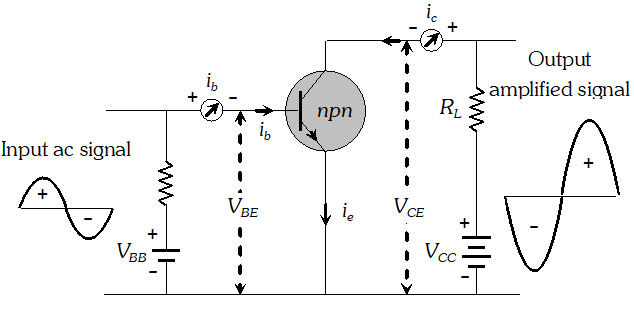$V_{B B}=$ Low tension battery for forward biasing base- emitter circuit $V_{C C}=$ High tension battery for reverse biasing collector-emitter circuit $V_{B E}=$ Potential difference between base to emitter $V_{c E}=$ Potential difference between collector to Emitter $R_{L}=$ Load resistance $i_{e}=$ emitter current, $i_{b}=$ Base current $i_{c}=$ collector Current Working : When no ac signal is applied, the potential difference between collector to emitter $V_{C E}$ is given by $V_{C E}=V_{C C}-i_{C} R_{L}$ …(i) When the input ac voltage signal is applied across the base-emitter circuit, it changes the base-emitter voltage $V_{B E}$ and hence the emitter current $i_{e}$ which, in turn, changes the collector current $i_{c}$ Consequently, the collector to emitter voltage $V_{C E}$ varies in accordance with equation (i). This variation in when the input signal is applied, appears as an amplified output. Phase relationship between input and output signals : Suppose the first half cycle of input voltage signal is positive. The positive half cycle of the input signal increases the forward biasing of the base-emitter circuit. Therefore and correspondingly the increases. As a result, the collector voltage decreases in accordance to the relation . A decrease in collector voltage means that the collector becomes less positive. Thus during the positive half cycle of the ac input voltage signal, the output voltage signal at the collector undergoes a negative half cycle. The negative half cycle of the input voltage opposes and hence reduces the forward biasing of the base-emitter circuit. Therefore and decreases. As a result, the collector voltage increases, i.e. collector becomes more positive. Thus during the negative half cycle of the ac input voltage signal, the output voltage signal undergoes a positive half cycle. Thus the output voltage signal is $180^{\circ}$ out of phase with the input voltage signal.
Q. Draw a circuit diagram to obtain the characteristics of a NPN transistor in common emitter configuration. Describe how you will obtain input and output characteristics. Give shape of the curves. Define the term (i) Output resistance and (ii) Current amplification factor
Ans. Circuit diagram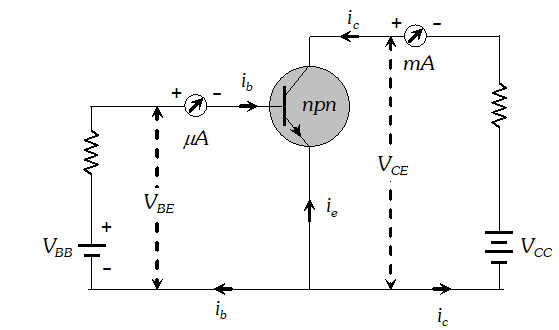Input characteristic : A graph between the base emitter voltage $V_{B E}$ and the base current at a constant collector emitter voltage $V_{C E}$ is called the input characteristic of the transistor. Output characteristic : A graph between the collector emitter voltage $V_{C E}$ and the collector current $i_{c}$ at a constant base current base current $\dot{l}_{b}$ is called the output characteristic of the transistor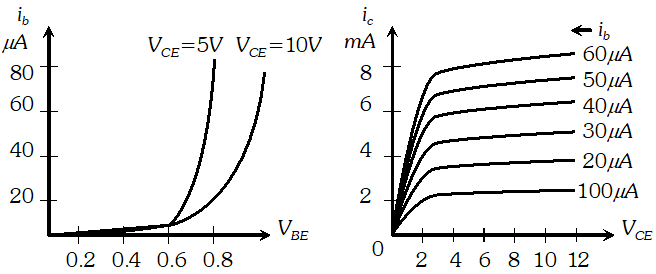Ans. Output resistance $\left(r_{0}\right):$ It is defined as the ratio of the collector voltage $\Delta V_{C E}$ to the corresponding change in collector current $\Delta i_{C}$ at constant base current $i_{b}$ i.e. $\quad r_{0}=\left[\frac{\Delta V_{C E}}{\Delta i_{C}}\right]_{i_{b}=\text { constant }}$ Current amplification factor $(\beta)$ : It is defined as the ratio of the change in collector current to the change in base current i.e. $\quad \beta=\left[\frac{\Delta i_{c}}{\Delta i_{b}}\right]_{V_{C B}=\mathrm{constant}}$
Q. A photodiode is fabricated from a semiconductor with band gap of 2.8 eV. Can it detect on wavelength of 6000 nm ? Justify
Ans. $\lambda=6000 n m=6 \times 10^{-6} \mathrm{m}$ $\therefore E=\frac{h c}{\lambda}=\frac{6.6 \times 10^{-34} \times 3 \times 10^{8}}{6 \times 10^{-6}}=3.3 \times 10^{-20} J$ $=\frac{3.3 \times 10^{-20}}{1.6 \times 10^{-19}}=0.206 e V$ As the energy of the photon is less than $E_{g}(=2.8 e V)$ of the semiconductor, so a wavelength of 6000 Å cannot be detected.
Q. A semiconductor has equal electron and hole concentration of $6 \times 10^{8} / \mathrm{m}^{3}$. On doping with certain impurity, electron concentration increases to $9 \times 10^{12} / m^{3}$ (i) Identify the new semiconductor obtained after doping (ii) Calculate the new hole concentration.
Ans. $n_{i}=6 \times 10^{8} / m^{3}, n_{e}=9 \times 10^{12} / m^{3}$ As $n_{e} n_{h}=n_{i}^{2}$ $\therefore n_{h}=\frac{n_{i}^{2}}{n_{e}}=\frac{\left(6 \times 10^{8}\right)^{2}}{9 \times 10^{12}}=4 \times 10^{4} / m^{3}$ As the new electron concentration is greater than the new hole concentration, so the new semiconductor is of n-type
Q. The input resistance of a transistor is 1000 $\Omega$. On changing its base current by $10 \mu \mathrm{A}$, the collector current increases by 2 mA. If a load resistance of 5 $K \Omega$is used in the circuit, calculate. (i) The current gain (ii) Voltage gain of the amplifier
Ans.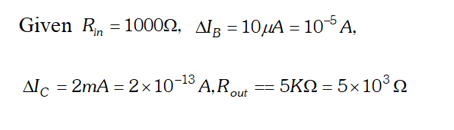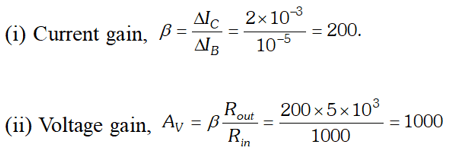Q. Suppose a pure Si crystal has $5 \times 10^{28}$ atoms $m^{-3}$ . It is doped by 1 ppm concentration of pentavalent As. Calculate the number of electrons and holes. Given that $n_{1}=1.5 \times 10^{16} \mathrm{m}^{-3}$ [NCERT]
Ans. Note that thermally generated electrons $\left(n_{i} \approx 10^{16} m^{-3}\right)$ are negligibly small as compared to those produced by doping. Therefore $n_{e}=N_{D}$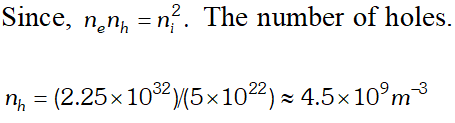Q.  Two amplifiers are connected one after the other in series (cascaded). The first amplifier has a voltage gain of 10 and the second has a voltage gain of 20. If the input signal is 0.01 V, calculate the output ac signal [NCERT]
Ans.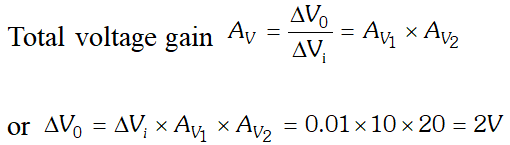Q. For a common emitter transistor amplifier, the audio signal voltage across the collector resistance of $2 k \Omega$is 2V. If the current amplification factor of the transistor is 100 calculate (i) Input signal voltage, (ii) Base current and (iii) power gain. Given that the value of the base resistance is 1 $k \Omega$
Ans. $R_{C}=2 k \Omega=2000 \Omega, R_{B}=1 k \Omega=1000 \Omega, \beta=100, V_{0}=2 V$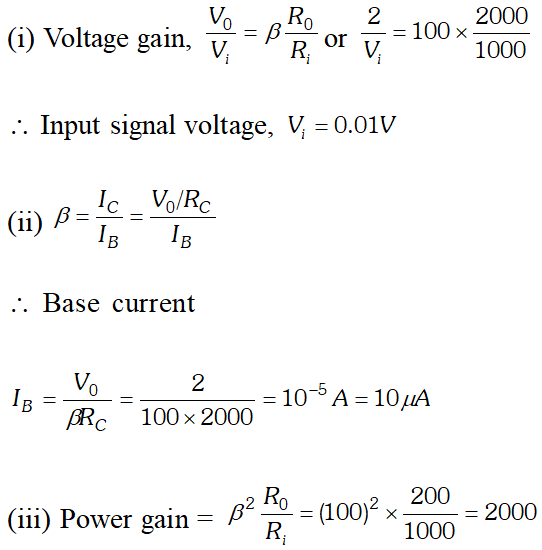Q. An n-p-n transistor in a common-emitter mode is used as a simple voltage amplifier with a collector current of 4 mA. The terminal of a 8 V battery is connected to the collector through a load resistance
Ans. For the given combination of gates, the truth table is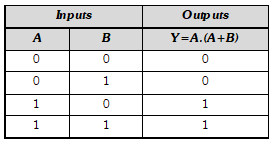Q. The output of an OR gate is connected to both the inputs of a NAND gate. Draw the logic circuit of this combination of gates and write its truth table.
Ans. Logic circuit of the combination is shown below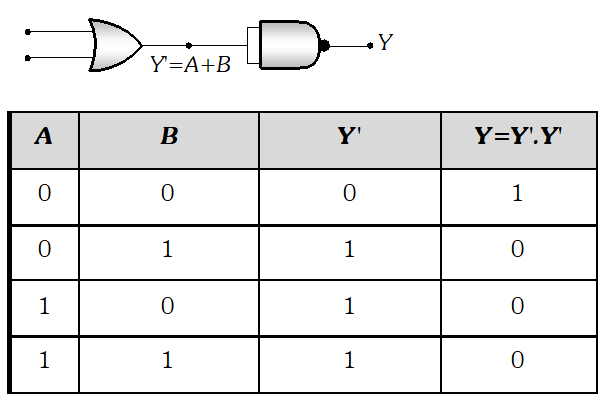Q. Identify the logic gates marked X, Y in the following figure. Write down the output at Z, when A=1, B=1 and A = 0, B = 1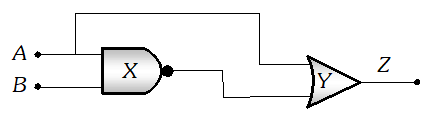Ans. X is NAND gate and Y is OR gate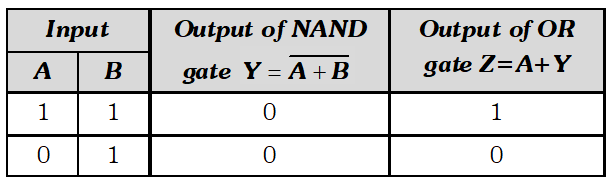Q.  In the figure below, circuit symbol of a logic gate and two input waveforms A and B are shown. (i) Name the logic gate (ii) Write its truth table (iii) Give the output waveform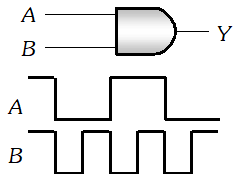Ans. (i) The logic gate is AND gate. (ii) Truth table of AND gate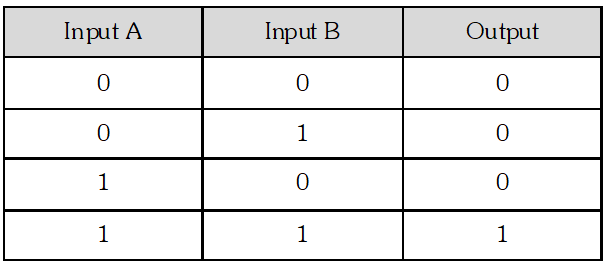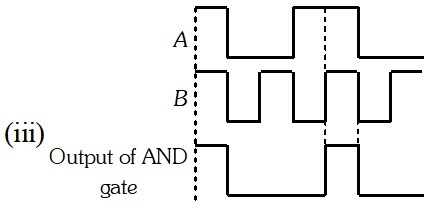Q. Give the logic symbol for an OR gate. Draw the output wave form for input wave forms A and B for this gate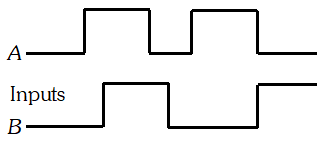Ans. Logic symbol of OR gate is given below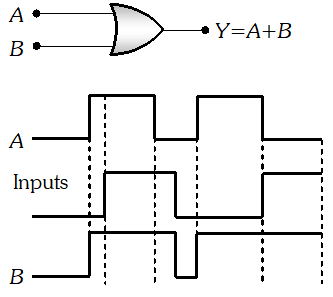Q. Two signals A and B shown in the given figure are used as two inputs of a NAND gate. Draw its output wave form. Give the logic symbol of NAND gate.
Ans. The output waveform of NAND gate is shown below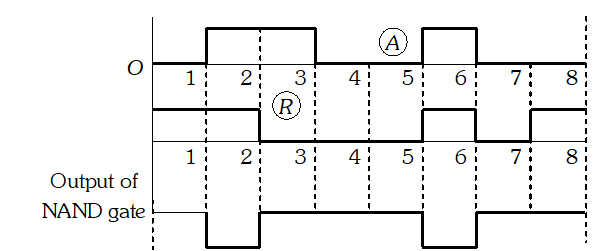Q. In the figure, circuit symbol of logic gate and input wave forms are given. Name the logic gate. Write its truth table and give the output wave form.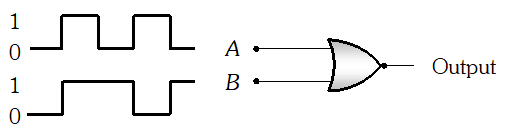Ans. The logic gate is NOR gate. The output waveform is shown below.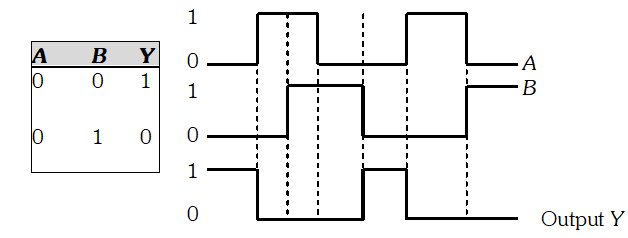Q. Give the symbol, and the truth table, of each of the two logic gates, obtained by using the two circuits, shown below.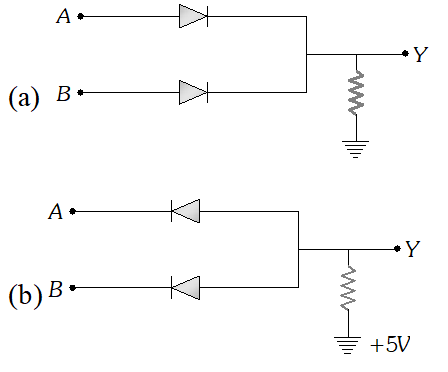Ans. (a) The given circuit represents OR gates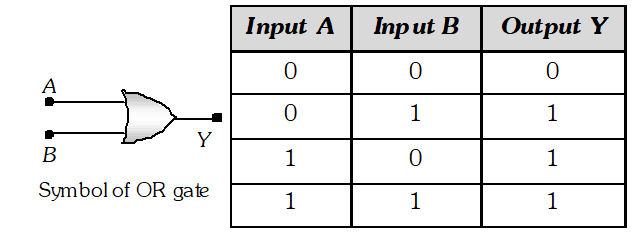(b) The given circuit represents AND gate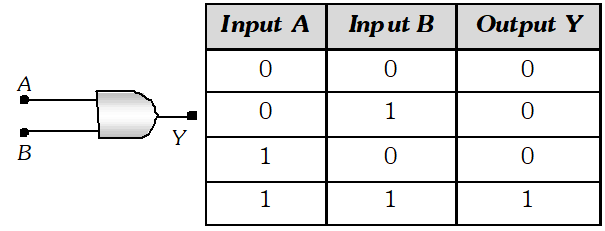Q. Show that a bubbled AND gate is equivalent to a NOR gates. Hence prove the identity [NCERT]
Ans. A bubbled AND gate is shown in figure (A) which is the combination of two NOT gates and one AND gate as shown in figure (B).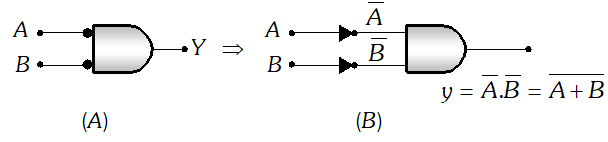The Boolean expression for output of this gate is $y=\bar{A} \bar{B}=\overline{A+B}$ (From De-Morgan’s theorem) As, $y=\overline{A+B}$ is the Boolean expression for NOR gate, hence bubbled, AND gate acts as NOR gate
eSaral provides you complete edge to prepare for Board and Competitive Exams like JEE, NEET, BITSAT, etc. We have transformed classroom in such a way that a student can study anytime anywhere. With the help of AI we have made the learning Personalized, adaptive and accessible for each and every one. Visit eSaral Website to download or view free study material for JEE & NEET. Also get to know about the strategies to Crack Exam in limited time period.